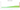# K

## Malawian kwacha

#### how has the value of the currency changed in the last year?## USD 1 = MWK 1,019.5

How much is10 US dollars worth inMalawian kwachas?
At the current exchange rate, 10 US dollars is worth 10,195 Malawian kwachas
How much is50 US dollars worth inMalawian kwachas?
At the current exchange rate, 50 US dollars is worth 50,975 Malawian kwachas
How much is100 US dollars worth inMalawian kwachas?
At the current exchange rate, 100 US dollars is worth 101,950 Malawian kwachas
How much is500 US dollars worth inMalawian kwachas?
At the current exchange rate, 500 US dollars is worth 509,750 Malawian kwachas
How much is2,000 US dollars worth inMalawian kwachas?
At the current exchange rate, 2,000 US dollars is worth 2,039,000 Malawian kwachas
How much is10 US dollars worth inMalawian kwachas?
At the current exchange rate, 10 US dollars is worth 10,195 Malawian kwachas
How much is50 US dollars worth inMalawian kwachas?
At the current exchange rate, 50 US dollars is worth 50,975 Malawian kwachas
How much is100 US dollars worth inMalawian kwachas?
At the current exchange rate, 100 US dollars is worth 101,950 Malawian kwachas
How much is500 US dollars worth inMalawian kwachas?
At the current exchange rate, 500 US dollars is worth 509,750 Malawian kwachas
How much is2,000 US dollars worth inMalawian kwachas?
At the current exchange rate, 2,000 US dollars is worth 2,039,000 Malawian kwachas